# NCERT Solutions Class 11 Mathematics Chapter 12 Introduction to Three Dimensional Geometry

## Chapter 12 Introduction to Three Dimensional Geometry Class 11 Mathematics NCERT Solutions

Class 11 Mathematics students should refer to the following NCERT questions with answers for Chapter 12 Introduction to Three Dimensional Geometry in Class 11. These NCERT Solutions with answers for Class 11 Mathematics will come in exams and help you to score good marks

### Chapter 12 Introduction to Three Dimensional Geometry NCERT Solutions Class 11 Mathematics

Exercise 12.1

Question 1: A point is on the x-axis. What are its y-coordinates and z-coordinates?

Answer :  If a point is on the x-axis, then its y-coordinates and z-coordinates are zero.

Question 2: A point is in the XZ-plane. What can you say about its y-coordinate?

Answer : If a point is in the XZ plane, then its y-coordinate is zero.

Question 3: Name the octants in which the following points lie:

(1, 2, 3), (4, –2, 3), (4, –2, –5), (4, 2, –5), (–4, 2, –5), (–4, 2, 5), (–3, –1, 6), (2, –4, –7)

Answer : The x-coordinate, y-coordinate, and z-coordinate of point (1, 2, 3) are all positive. Therefore, this point lies in octant I.

The x-coordinate, y-coordinate, and z-coordinate of point (4, –2, 3) are positive, negative, and positive respectively. Therefore, this point lies in octant IV.

The x-coordinate, y-coordinate, and z-coordinate of point (4, –2, –5) are positive, negative, and negative respectively. Therefore, this point lies in octant VIII.

The x-coordinate, y-coordinate, and z-coordinate of point (4, 2, –5) are positive, positive, and negative respectively. Therefore, this point lies in octant V.

The x-coordinate, y-coordinate, and z-coordinate of point (–4, 2, –5) are negative, positive, and negative respectively. Therefore, this point lies in octant VI.

The x-coordinate, y-coordinate, and z-coordinate of point (–4, 2, 5) are negative, positive, and positive respectively. Therefore, this point lies in octant II.

The x-coordinate, y-coordinate, and z-coordinate of point (–3, –1, 6) are negative, negative, and positive respectively. Therefore, this point lies in octant III.

The x-coordinate, y-coordinate, and z-coordinate of point (2, –4, –7) are positive, negative, and negative respectively. Therefore, this point lies in octant VIII.

4. Fill in the blanks:
(i) The x-axis and y-axis taken together determine a plane known as______.
(ii) The coordinates of points in the XY-plane are of the form ______.
(iii) Coordinate planes divide the space into ______ octants.

(i) The x-axis and y-axis taken together determine a plane known as XY-plane.

(ii) The coordinates of points in the XY-plane are of the form (x, y, 0) .

(iii) Coordinate planes divide the space into eight octants.

Exercise 12.2

1. Find the distance between the following pairs of points:
(i) (2, 3, 5) and (4, 3, 1)
(ii) (–3, 7, 2) and (2, 4, –1)
(iii) (–1, 3, –4) and (1, –3, 4)
(iv) (2, –1, 3) and (–2, 1, 3)

The distance between points P(x1, y1, z1) and P(x2, y2, z2) is given by(i) Distance between points (2, 3, 5) and (4, 3, 1)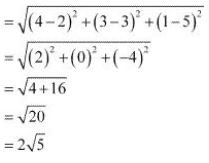(ii) Distance between points (-3, 7, 2) and (2, 4, -1)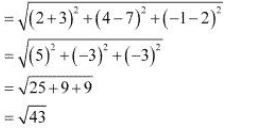(iv) Distance between points (2, -1, 3) and (-2, 1, 3)2. Show that the points (–2, 3, 5), (1, 2, 3) and (7, 0, –1) are collinear.

Let points (–2, 3, 5), (1, 2, 3), and (7, 0, –1) be denoted by P, Q, and R respectively.

Points P, Q, and R are collinear if they lie on a line.Here, PQ + QR = √14 + 2√14 = 3√14 = PR

Hence, points P(-2, 3, 5), Q(1, 2, 3), and R(7, 0, -1) are collinear.

3. Verify the following:
(i) (0, 7, –10), (1, 6, –6) and (4, 9, –6) are the vertices of an isosceles triangle.
(ii) (0, 7, 10), (–1, 6, 6) and (–4, 9, 6) are the vertices of a right angled triangle.

(iii) (–1, 2, 1), (1, –2, 5), (4, –7, 8) and (2, –3, 4) are the vertices of a parallelogram.

(i) Let points (0, 7, -10), (1, 6, -6), and (4, 9, -6) be denoted by A, B, and C respectively.Here, AB = BC ≠ CA

Thus, the given points are the vertices of an isosceles triangle.

(ii) Let (0, 7, 10), (–1, 6, 6), and (–4, 9, 6) be denoted by A, B, and C respectively.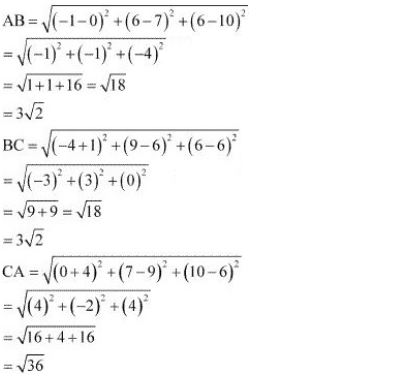Now, AB2 + BC2 = (3√2)2 + (3√2)2 = 18 + 18 = 36 = AC2.

Therefore, by Pythagoras theorem, ABC is a right triangle.

Hence, the given points are the vertices of a right - angled triangle.

(iii) Let (-1, 2, 1), (1, -2, 5), (4, -7, 8), and (2, -3, 4) be denoted by A, B, C, and D respectively.Here, AB = CD = 6, BC = AD = √43

Hence, the opposite sides of quadrilateral ABCD, whose vertices are taken in order, are equal.

Therefore, ABCD is a parallelogram.

Hence, the given points are the vertices of a parallelogram.

4. Find the equation of the set of points which are equidistant from the points (1, 2, 3) and (3, 2, –1).

Let P (x, y, z) be the point that is equidistant from points A(1, 2, 3) and B(3, 2, –1).

Accordingly, PA = PB

⇒ PA2 = PB2

⇒ (x - 1)2 + (y - 2)2 + (z - 3)2 = (x -3)2 + (y - 2)2 + (z + 1)2

⇒ x2 - 2x + 1 + y2 - 4y + 4 +z2 - 6z + 9 = x2 - 6x + 9 + y2 - 4y + 4 + z2 + 2z + 1

⇒ -2x - 4y - 6z + 14 = -6x - 4y + 2z + 14

⇒ -2x - 6z + 6x - 2z = 0

⇒ 4x - 8z = 0

⇒ x - 2z = 0

Thus, the required equation is x - 2z = 0.

5. Find the equation of the set of points P, the sum of whose distances from A (4, 0, 0) and B (–4, 0, 0) is equal to 10.

Solution

Let the coordinates of P be (x, y, z).

The coordinates of points A and B are (4, 0, 0) and (–4, 0, 0) respectively.

It is given that PA + PB = 10.On squaring both sides again, we obtain

25 (x2 + 8x + 16 + y2 + z2) = 625 + 16x2 + 200x

⇒ 25x2 + 200x + 400 + 25y2 + 25z2 = 625 + 16x2 + 200x

⇒ 9x2 + 25y2 + 25z2 – 225 = 0

Thus, the required equation is 9x2 + 25y2 + 25z2 – 225 = 0.

Exercise 12.3

1. Find the coordinates of the point which divides the line segment joining the points (–2, 3, 5) and (1, –4, 6) in the ratio (i) 2:3 internally, (ii) 2:3 externally.

(i) The coordinates of point R that divides the line segment joining points P (x1, y1, z1) and Q (x2, y2, z2) internally in the ratio m: n arei.e., x = -8, y = 17, and z = 3

Thus, the coordinates of the required point are (-8, 17, 3).

2. Given that P (3, 2, –4), Q (5, 4, –6) and R (9, 8, –10) are collinear. Find the ratio in which Q divides PR.

Let point Q (5, 4, –6) divide the line segment joining points P (3, 2, –4) and R (9, 8, –10) in the ratio k:1.

Therefore, by section formula,⇒ 9k + 3 = 5k + 5

⇒ 4k = 2

⇒ k = 2/4 = 1/2

Thus, point Q divides PR in the ratio 1 : 2.

3. Find the ratio in which the YZ-plane divides the line segment formed by joining the points (–2, 4, 7) and (3, –5, 8).

Solution

Let the YZ planedivide the line segment joining points (–2, 4, 7) and (3, –5, 8) in the ratio k:1.

Hence, by section formula, the coordinates of point of intersection are given by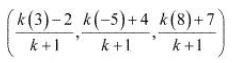On the YZ plane, the x - coordinate of any point is zero.

(3k - 2)/(k + 1) = 0

⇒ 3k -2 = 0

⇒ k = 2/3

Thus, the YZ plane divides the line segment formed by joining the given points in the ratio 2 : 3.

4. Using section formula, show that the points A (2, –3, 4), B (–1, 2, 1) and  C(0, 1/3, 2) are collinear.

The given points are A (2, –3, 4), B (–1, 2, 1), and C(0, 1/3, 2). .

Let P be a point that divides AB in the ratio k:1.

Hence, by section formula, the coordinates of P are given by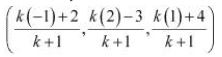Now, we find the value of k at which point P coincides with point C.

By taking (-k + 2)/(k + 1) = 0 , we obtain k = 2.

For k = 2, the coordinates of point P are (0, 1/3, 2).

i.e, C(0, 1/3, 2) is a point that divides AB externally in the ratio 2 : 1 and is the same as point P .

Hence, points A, B, and C are collinear.

5. Find the coordinates of the points which trisect the line segment joining the points P (4, 2, –6) and Q (10, –16, 6).

Let A and B be the points that trisect the line segment joining points P (4, 2, –6) and Q (10, –16, 6)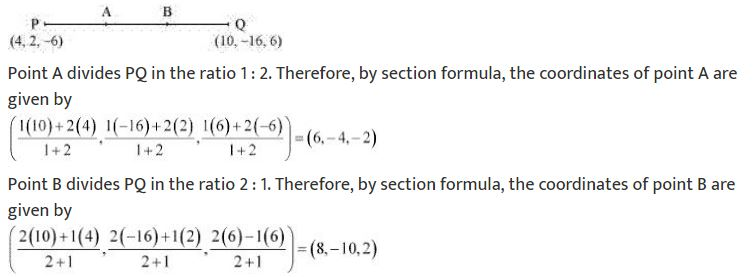Thus, (6, -4, -2) and (8, -10, 2) are the points that trisect the line segment joining points P(4, 2, -6) and Q(10, -16, 6).

Miscellaneous Exercise

1. Three vertices of a parallelogram ABCD are A (3, –1, 2), B (1, 2, –4) andC (–1, 1, 2). Find the coordinates of the fourth vertex.

The three vertices of a parallelogram ABCD are given as A (3, –1, 2), B (1, 2, –4), and C (–1, 1, 2). Let the coordinates of the fourth vertex be D (x,y,z).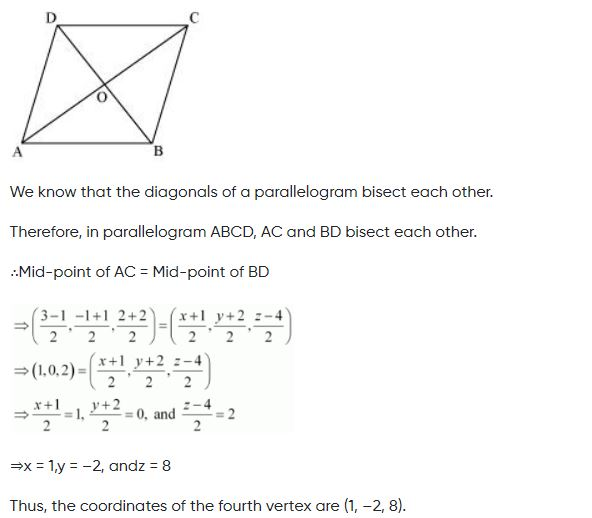2. Find the lengths of the medians of the triangle with vertices A (0, 0, 6), B (0, 4, 0) and (6, 0, 0).

Let AD, BE, and CF be the medians of the given triangle ABC.3. If the origin is the centroid of the triangle PQR with vertices P (2a, 2, 6), Q (–4, 3b, –10) and R (8, 14, 2c), then find the values ofa,b andc.It is known that the coordinates of the centroid of the triangle, whose vertices are (x1,y1,z1),  (x2,y2,z2) and (x3,y3,z3), are4. Find the coordinates of a point ony-axis which are at a distance of 52 from the point P (3, –2, 5).

If a point is on the y-axis, then x-coordinate and the z-coordinate of the point are zero.

Let A (0, b, 0) be the point on the y-axis at a distance of AP = 5√2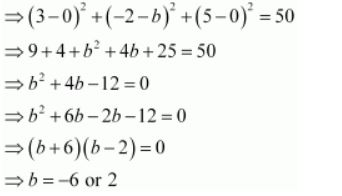Thus, the coordinates of the required points are (0, 2, 0) and (0, –6, 0).

5. A point R withx-coordinate 4 lies on the line segment joining the pointsP (2, –3, 4) and Q (8, 0, 10). Find the coordinates of the point R.

[Hint suppose R divides PQ in the ratio k: 1. The coordinates of the point R are given by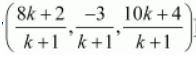The coordinates of points P and Q are given as P (2, –3, 4) and Q (8, 0, 10).

Let R divide line segment PQ in the ratio k:1.

Hence, by section formula, the coordinates of point R are given by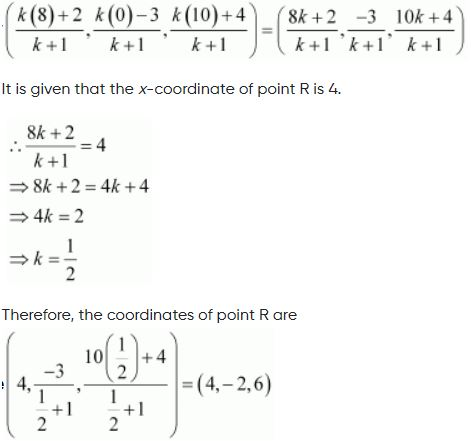6. If A and B be the points (3, 4, 5) and (–1, 3, –7), respectively, find the equation of the set of points P such that PA2 + PB2 =k2, wherek is a constant.

The coordinates of points A and B are given as (3, 4, 5) and (–1, 3, –7) respectively.

Let the coordinates of point P be (xyz).

On using distance formula, we obtain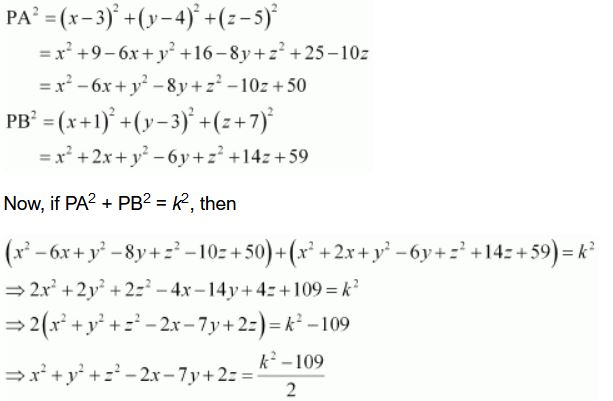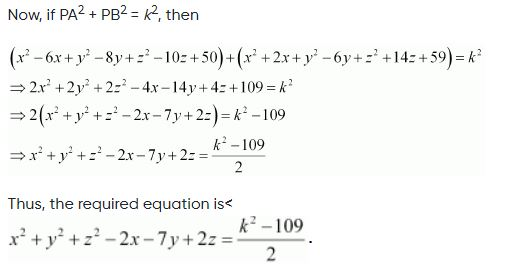NCERT Solutions Class 11 Mathematics Chapter 1 Sets
 NCERT Solutions Class 11 Mathematics Chapter 2 Relations and Functions
 NCERT Solutions Class 11 Mathematics Chapter 3 Trigonometric Functions
 NCERT Solutions Class 11 Mathematics Chapter 4 Principle of Mathematical Induction
 NCERT Solutions Class 11 Mathematics Chapter 5 Complex Numbers and Quadratic Equations
 NCERT Solutions Class 11 Mathematics Chapter 6 Linear Inequalities
 NCERT Solutions Class 11 Mathematics Chapter 7 Permutations and Combinations
 NCERT Solutions Class 11 Mathematics Chapter 8 Binomial Theorem
 NCERT Solutions Class 11 Mathematics Chapter 9 Sequences and Series
 NCERT Solutions Class 11 Mathematics Chapter 10 Straight Lines
 NCERT Solutions Class 11 Mathematics Chapter 11 Conic Sections
 NCERT Solutions Class 11 Mathematics Chapter 12 Introduction to Three Dimensional Geometry
 NCERT Solutions Class 11 Mathematics Chapter 13 Limits and Derivatives
 NCERT Solutions Class 11 Mathematics Chapter 14 Mathematical Reasoning
 NCERT Solutions Class 11 Mathematics Chapter 15 Statistics
 NCERT Solutions Class 11 Mathematics Chapter 16 Probability

## More Study Material

Tags

### NCERT Solutions Class 11 Mathematics Chapter 12 Introduction to Three Dimensional Geometry

NCERT Solutions Class 11 Mathematics Chapter 12 Introduction to Three Dimensional Geometry is available on our website www.studiestoday.com for free download in Pdf. You can read the solutions to all questions given in your Class 11 Mathematics textbook online or you can easily download them in pdf.

### Chapter 12 Introduction to Three Dimensional Geometry Class 11 Mathematics NCERT Solutions

The Class 11 Mathematics NCERT Solutions Chapter 12 Introduction to Three Dimensional Geometry are designed in a way that will help to improve the overall understanding of students. The answers to each question in Chapter 12 Introduction to Three Dimensional Geometry of Mathematics Class 11 has been designed based on the latest syllabus released for the current year. We have also provided detailed explanations for all difficult topics in Chapter 12 Introduction to Three Dimensional Geometry Class 11 chapter of Mathematics so that it can be easier for students to understand all answers.

#### NCERT Solutions Chapter 12 Introduction to Three Dimensional Geometry Class 11 Mathematics

Class 11 Mathematics NCERT Solutions Chapter 12 Introduction to Three Dimensional Geometry is a really good source using which the students can get more marks in exams. The same questions will be coming in your Class 11 Mathematics exam. Learn the Chapter 12 Introduction to Three Dimensional Geometry questions and answers daily to get a higher score. Chapter 12 Introduction to Three Dimensional Geometry of your Mathematics textbook has a lot of questions at the end of chapter to test the students understanding of the concepts taught in the chapter. Students have to solve the questions and refer to the step-by-step solutions provided by Mathematics teachers on studiestoday to get better problem-solving skills.

#### Chapter 12 Introduction to Three Dimensional Geometry Class 11 NCERT Solution Mathematics

These solutions of Chapter 12 Introduction to Three Dimensional Geometry NCERT Questions given in your textbook for Class 11 Mathematics have been designed to help students understand the difficult topics of Mathematics in an easy manner. These will also help to build a strong foundation in the Mathematics. There is a combination of theoretical and practical questions relating to all chapters in Mathematics to check the overall learning of the students of Class 11.

#### Class 11 NCERT Solution Mathematics Chapter 12 Introduction to Three Dimensional Geometry

NCERT Solutions Class 11 Mathematics Chapter 12 Introduction to Three Dimensional Geometry detailed answers are given with the objective of helping students compare their answers with the example. NCERT solutions for Class 11 Mathematics provide a strong foundation for every chapter. They ensure a smooth and easy knowledge of Revision notes for Class 11 Mathematics. As suggested by the HRD ministry, they will perform a major role in JEE. Students can easily download these solutions and use them to prepare for upcoming exams and also go through the Question Papers for Class 11 Mathematics to clarify all doubts

Where can I download latest NCERT Solutions for Class 11 Mathematics Chapter 12 Introduction to Three Dimensional Geometry

You can download the NCERT Solutions for Class 11 Mathematics Chapter 12 Introduction to Three Dimensional Geometry for latest session from StudiesToday.com

Can I download the NCERT Solutions of Class 11 Mathematics Chapter 12 Introduction to Three Dimensional Geometry in Pdf

Yes, you can click on the link above and download NCERT Solutions in PDFs for Class 11 for Mathematics Chapter 12 Introduction to Three Dimensional Geometry

Are the Class 11 Mathematics Chapter 12 Introduction to Three Dimensional Geometry NCERT Solutions available for the latest session

Yes, the NCERT Solutions issued for Class 11 Mathematics Chapter 12 Introduction to Three Dimensional Geometry have been made available here for latest academic session

How can I download the Chapter 12 Introduction to Three Dimensional Geometry Class 11 Mathematics NCERT Solutions

You can easily access the links above and download the Chapter 12 Introduction to Three Dimensional Geometry Class 11 NCERT Solutions Mathematics for each chapter

Is there any charge for the NCERT Solutions for Class 11 Mathematics Chapter 12 Introduction to Three Dimensional Geometry

There is no charge for the NCERT Solutions for Class 11 Mathematics Chapter 12 Introduction to Three Dimensional Geometry you can download everything free

How can I improve my scores by reading NCERT Solutions in Class 11 Mathematics Chapter 12 Introduction to Three Dimensional Geometry

Regular revision of NCERT Solutions given on studiestoday for Class 11 subject Mathematics Chapter 12 Introduction to Three Dimensional Geometry can help you to score better marks in exams

Are there any websites that offer free NCERT solutions for Chapter 12 Introduction to Three Dimensional Geometry Class 11 Mathematics

Yes, studiestoday.com provides all latest NCERT Chapter 12 Introduction to Three Dimensional Geometry Class 11 Mathematics solutions based on the latest books for the current academic session

Can NCERT solutions for Class 11 Mathematics Chapter 12 Introduction to Three Dimensional Geometry be accessed on mobile devices

Yes, studiestoday provides NCERT solutions for Chapter 12 Introduction to Three Dimensional Geometry Class 11 Mathematics in mobile-friendly format and can be accessed on smartphones and tablets.

Are NCERT solutions for Class 11 Chapter 12 Introduction to Three Dimensional Geometry Mathematics available in multiple languages

Yes, NCERT solutions for Class 11 Chapter 12 Introduction to Three Dimensional Geometry Mathematics are available in multiple languages, including English, Hindi